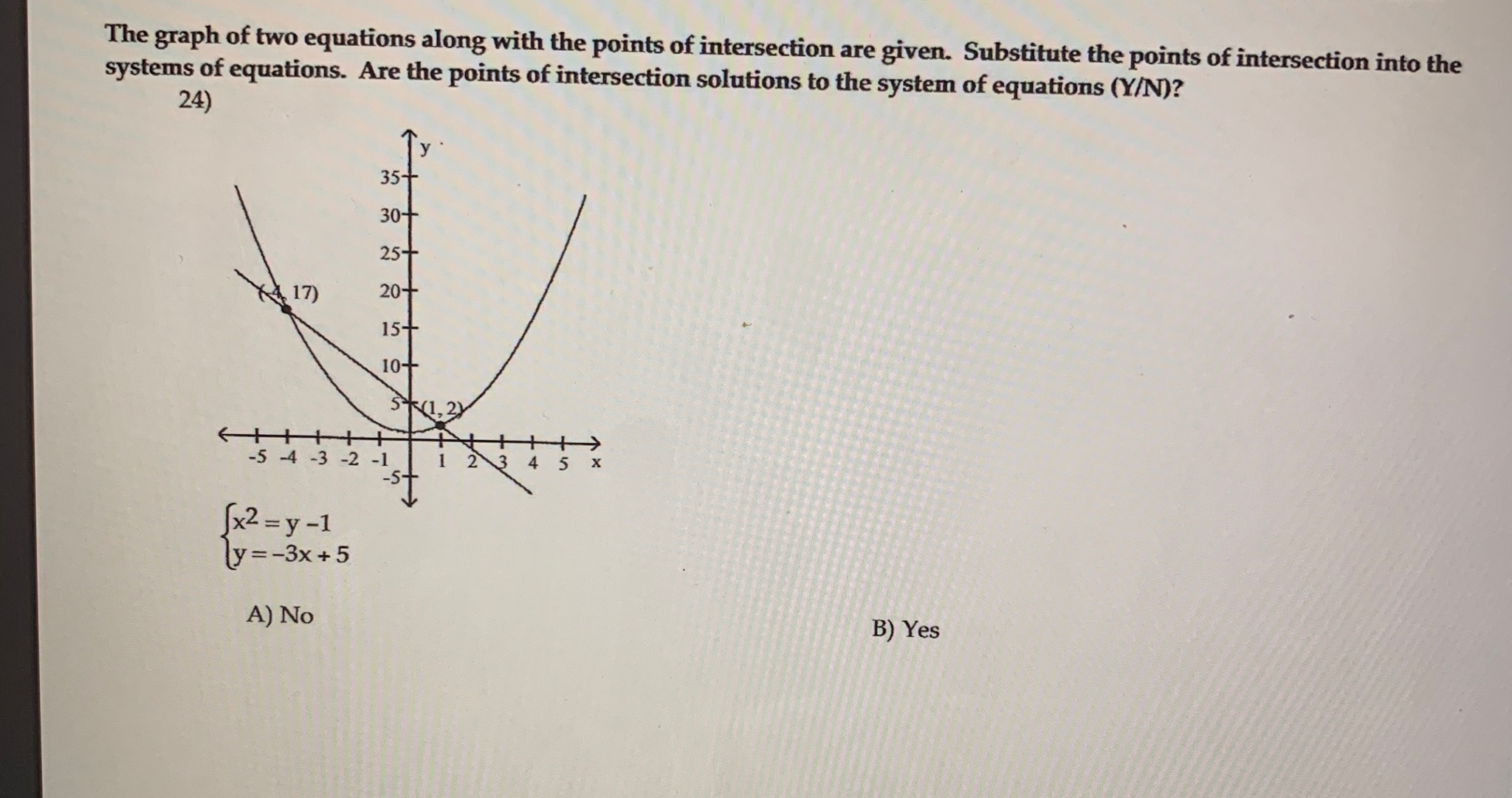# The graph of two equations along with the points of intersection are given. Substitute the points of intersection into the systems of equations. Are the points of intersection solutions to the system of equations (Y/N)? 24) 35- 30+ 25+ 17) 20+ 15+ 10+ 5*1, 2) -5 -4 -3 -2 -1 х x2 = y -1 ly =-3x+5 A) No B) Yes

Questionhelp_outlineImage TranscriptioncloseThe graph of two equations along with the points of intersection are given. Substitute the points of intersection into the systems of equations. Are the points of intersection solutions to the system of equations (Y/N)? 24) 35- 30+ 25+ 17) 20+ 15+ 10+ 5*1, 2) -5 -4 -3 -2 -1 х x2 = y -1 ly =-3x+5 A) No B) Yes fullscreen

### Want to see this answer and more?

Experts are waiting 24/7 to provide step-by-step solutions in as fast as 30 minutes!*

*Response times may vary by subject and question complexity. Median response time is 34 minutes for paid subscribers and may be longer for promotional offers.
Tagged in
Math
Algebra

### Equations and In-equations